# Graphical Modelling in Simile : System Dynamics

## System Dynamics modelling in Simile

A model is made up of compartments, representing quantities of material, and flows, representing movements between compartments. Where material moves in or out of the area of interest, a flow ends in a cloud. A flow has a bowtie, representing a valve or pump that sets its rate. Flows are calculated from compartment levels, and variables can be used for intermediate results, for constants, or for getting data into or our of the model. Influences indicate which component values affect which others.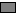compartment An amount of some substance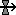flow A process moving a substance between compartments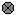variable A constant or variable quantity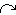influence Represents the fact that one quantity is used to calculate another

#Compartment

## How to add a Compartment symbol

See Adding node-type elements. Note that you can add a compartment on top of a cloud, in which case the cloud becomes a compartment.

## Interpretation

The compartment symbol is used to represent a quantitative state variable. Notionally, we think of a compartment as containing an amount of some substance, though it can be used in other situations where we want to represent the concept of state.

The informal interpretation of a compartment in System Dynamics modelling is that it represents a real, physical compartment that can contain some substance, just like a tank holds water. The compartment requires to be given an initial value - how much water does the tank hold at the start of the simulation? - and we need to construct flows in and out of the compartment so that the amount it holds can change over time.

This interpretation is fine to begin with, but must not be taken too literally. A compartment in System Dynamics modelling is, mathematically-speaking, a state variable: i.e. it is a variable whose behaviour is described by a differential (or difference) equation. And, unlike real, physical compartments, a compartment in System Dynamics:

• can go negative (if the flows out are greater than the flows in, when the compartment gets to zero);
• has infinite capacity (can go on increasing indefinitely);
• cannot contain multiple substances. A real tank can contain both water and oil, but in System Dynamics modelling we would need a separate compartment for each one. However Simile allows a compartment to be an array with the dimensions of an enumerated type -- this could be used to model a single tank containing multiple substances.
• can represent some state that does not correspond to the amount of a substance (such as the height of a tree, the area of land, the time when some event happened, or the x co-ordinate of a moving object).

## Rules

• You should not draw an influence arrow to a compartment, except for the special case of initialising it from other model variables. The behaviour of a compartment is determined solely by the net flows in and out of it. Its value at any point of time is found by incrementing or decrementing its value from the previous time step with the net inflow. But when you draw an influence arrow to a model element, you are saying that its value is calculated directly from the influencing variable, and that is incompatible with an approach base on adding or subtracting something from its previous value.
• If you do draw one or more influence arrows to a compartment to initialise it in terms of other model variables, then those variables should be static (i.e. not time-varying).
• If two compartments are connected by a flow arrow, then the two compartments should represent the same substance, and should have the same units.

#Flow arrow

## Interpretation

The flow arrow is used to specify a term contributing to the rate of change of a compartment. If the flow arrow enters a compartment, it specifies a positive contribution to the rate of change of that compartment. If it leaves the compartment, it specifies a negative contribution to the rate of change.

The information on the flows entering and leaving each compartment is used to calculate the net rate of change of the compartment. The net rate of change is the sum of all the inflow values minus the sum of all the outflow values. The net rate of change is in turn used to calculate the change in the value of the compartment.

If your model needs to keep track of changes in the amount of a substance but you are not interested in where it comes from or goes to, your flow may start or finish on a blank part of the model diagram. In this case a "cloud" will be drawn at the end point, indicating that the amount of substance there plays no role in the model. Each cloud may only have one flow connected to it.

Influences to and from a flow are attached to a "bowtie" (or "valve") symbol which is positioned on the flow. This represents the point that controls the rate of the flow.

In most respects, a flow is treated just like a variable. You can use the full range of the equation language when you enter an equation for the flow, just as you can do for a variable. You can have influence arrows going from it to other parts of the model, again just like a variable. The two differences are that:

• a flow is the only way you can express a rate of change term for a compartment; and
• a flow cannot be a "file parameter".

## Rules

• A flow arrow can only be drawn into and/or out of a compartment.
• Influence arrows can be drawn to and from flows.
• The units for a flow value must be the units of the corresponding compartment(s) that it is linked to, per unit of time. For example, if you have a flow going into a compartment whose units are kg, and the unit of time for the model is a year, then the units for the flow must be kg/year.
• It is quite legitimate to have an influence arrow going from one flow to another. You might wish to do this if one flow is proportional to another, or if the two flows are in different submodels.

#Variable

## Interpretation

A variable is used to hold one or more values. The value or values come from a mathematical expression. The expression may simply be a number, or it may be a complex mathematical expression involving various variables, operators (such as + and -), functions (such as log or square root), and conditional elements. The value of a variable may vary during the course of a simulation, if it is calculated from other parts of the model that change over time, or it may be constant.

The term "variable" is used to refer to a specific type of model element. This single element can be used for a wide variety of purposes, each of which is referred to in a different way by some modellers. There is rich potential for confusion here, so the following table sets out the correspondence between how a Simile variable is used in a model, and how a modeller would interpret that use. (In case you are wondering why we don't have a number of model elements, one for each type of use: the answer is that this would lead to an unnecessary proliferation of element types. Also, you might wish to change the role of a variable as you build up a model, and you would not want to have to keep on deleting one symbol and replacing it by another.)

 Modelling use Set-up of "variable"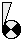Parameter (a coefficient in an equation): e.g. the reproductive rate per individual animal. Could also be a site constant: e.g. elevation above sea level. Its value will remain constant throughout a simulation run. No influence arrows pointing to it. One or more influence arrows pointing from it. Value is a numeric constant or value is not supplied and "Fixed parameter" radio button is selected.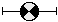Input lever: a slider control can be generated for each such variable, and the user can modify its value during the course of a simulation run by moving the slider left or right. No influence arrows pointing to it. One or more influence arrows pointing from it. Value is a numeric constant (representing initial slider position). "Variable parameter" radio button is selected. Exogenous variable: this is a variable whose value changes during a simulation run, and which influences the value of other variables, but which is not itself influenced by other variables. Typically used for climatic inputs, such as temperature or rainfall. No influence arrows pointing to it. One or more influence arrows pointing from it. Expression is some function of simulation time (i.e. involves the built-in function time). Intermediate variable One or more influence arrows pointing to it. One or more influence arrows pointing from it Output variable: typically, this is used to report on some aspect of model behaviour (e.g. the ratio of two compartments). One or more influence arrows point to it. No influence arrows pointing from it. Attribute of an object: there is only sense in doing this if the variable is inside a multiple-instance submodel, with different instances having different values. E.g. the x-coordinate or the species type of each of many trees. No influence arrows pointing to it. No influence arrows pointing from it.

## Rules

• A variable symbol may have zero or more influence arrows pointing to it.
• A variable cannot have influence arrows pointing to it if it is a "file parameter".
• A variable symbol may have zero or more influence arrows pointing from it.
• A variable may not have other kinds of arrow pointing to or from it.

#Influence arrow

## Interpretation

To say that "A influences B" (i.e. to draw an influence arrow from A to B) means that A is used to calculate a value for B: in other words, the equation for calculating B will include A.

## Rules

You can drag an influence arrow from and to most model elements. The exceptions and special cases are noted here below. Note that if you try to drag an influence arrow to a model element that cannot receive one, then it turns blue instead of green, and you will not be able to connect them together. You can store comments associated with an influence arrow by double-clicking the arrow.

###Compartments

• Elements that influence a compartment are used only to calculate the initial value of the compartment. Thereafter, the value of the compartment is calculated by adding the flows in and subtracting the flows out. It is not common therefore to draw an influence arrow to a compartment, though it is possible (in order to calculate the initial value). For example, although one may informally say "water temperature influences fish population size", in the model, temperature must actually influence one of the processes (reproduction, death and so forth) which change the fish population size. The influence arrow from the temperature variable must therefore point to one (or more) of the flows in or out , and not to the compartment representing fish population size itself.

###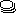Submodels

• When working with submodels, you may find that an input parameter (A) in one submodel actually corresponds to a variable (B) in another, especially when the two submodels were developed separately, then later brought together. In order to get rid of the duplication, draw an influence arrow from B to the influence arrow from A. This is the only circumstance in which it is possible to draw one influence arrow pointing to another. Input parameter A will disappear, and the influence arrows will be re-drawn to show B directly influencing the element previously influenced by A.
• You can drag an influence arrow to a submodel boundary, from either inside or outside the submodel. This has no meaningful modelling function, but acts as a temporary placeholder to the influence arrow until it is connected to an element on the other side of the boundary. In order to do this, drag an influence arrow from the head of the existing arrow to the desired element.
• You cannot drag an influence arrow from a submodel boundary, other than to complete an influence arrow drawn to the submodel boundary (as described in the previous bullet point).

###Influence and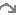role arrows

• You cannot drag an influence to a role arrow or to another influence arrow.
• If an influence shares part of its route with one or more role arrows (in either direction), then the normal interpretation is that the values it supplies will only be those from the base model instances in the corresponding roles, or from the association model instances for which the instance containing the destination is in the appropriate role. The different interpretations are selected by prefixing the caption of the source component (or suffixing if going from association to base) with the caption of the relevant role. As of Simile v6, the interpretations can be enabled or disabled by checkboxes in the influence properties dialogue. Also the interpretation that would apply if the role arrow were not present, i.e., get values from all source model instances, is also available and can also be enabled or disabled.
• In Simile v6.1, an alternative interpretation is provided for influences within multiple-instance submodels -- the ability to get values from all instances, as if the destination were outside the submodel, rather than just from the current instance. Furthermore, for influences within special-purpose submodels, there are interpretations that provide values from just a special subset of other instances, e.g., neighbour instances. Again, any of these (as well as the default interpretation) can be enabled or disabled from the properties dialogue.
• If an interpretation is enabled that gets values from other instances than the current one, the influence arrow will be decorated with an oblique stack that it passes through, indicating that it carries values between different instances of its submodel.
• To invoke the property dialogue box for an influence arrow, double-click on the arrow, or right-click on it and select "Properties..." from the context menu.

###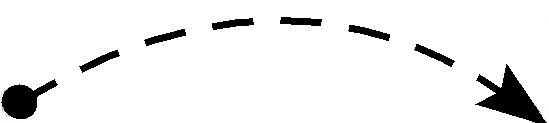Calculation order

An influence arrow indicates that the value of one component is used in calculating the value of another. So it puts a constraint on the order in which the two values can be calculated each time step; the one at the head must be done after the one at the tail.

This means that if it is possible to get from a model component around a loop of influence arrows back to the same component, the model cannot be executed, because no ordering of the calculations can satisfy all the constraints. The problem is called circularity. Usually this problem indicates that a certain variable in the model should in fact be a compartment, and an influence that connects to it should instead connect to a flow going to it. But there are some circumstances in which circular influences are OK.

• Influence arrows have one further property that can be set. The property is "Use values made in same time step". The property indicates how the ambiguity associated with a circular loop of influences is to be resolved.
• Normally, the value of the component at the end of an influence is calculated after that at its start in each time step. Selecting "Use values..." removes this constraint, and shows this by drawing the arrow dashed instead of solid.
• This may be used in combination with the iteration symbol to implement many iterative methods.
• You can also use this property to create a model process that iterates a fixed number of times and stores all its intermediate results in an array. In this case you do not need the iteration symbol. If you have two components in different fixed-membership submodels, and each has the same number of instances, then they can each have an influence to the other provided one of the influences has the "Use values made in same time step" property, and the equation of the component at the end of this influence uses only values of the other component with indices lower than its own. Subject to a similar restriction it is also possible to specify iteration between a base submodel and its association submodels.

There are two functions in the equation language, "last()" and "sofar()", which also allow a model with a circular chain of influences to execute. Putting "last()" around an expression means that its value at the end of the last time step is to be used, so the component using it does not have to be evaluated after it each time step. Putting "sofar()" round a value has the same effect as selecting the "Use values..." property described above for the influences used by that value; it is used to resolve circularity problems involving intermediate variables, where there is no influence arrow on which the property could be set.